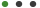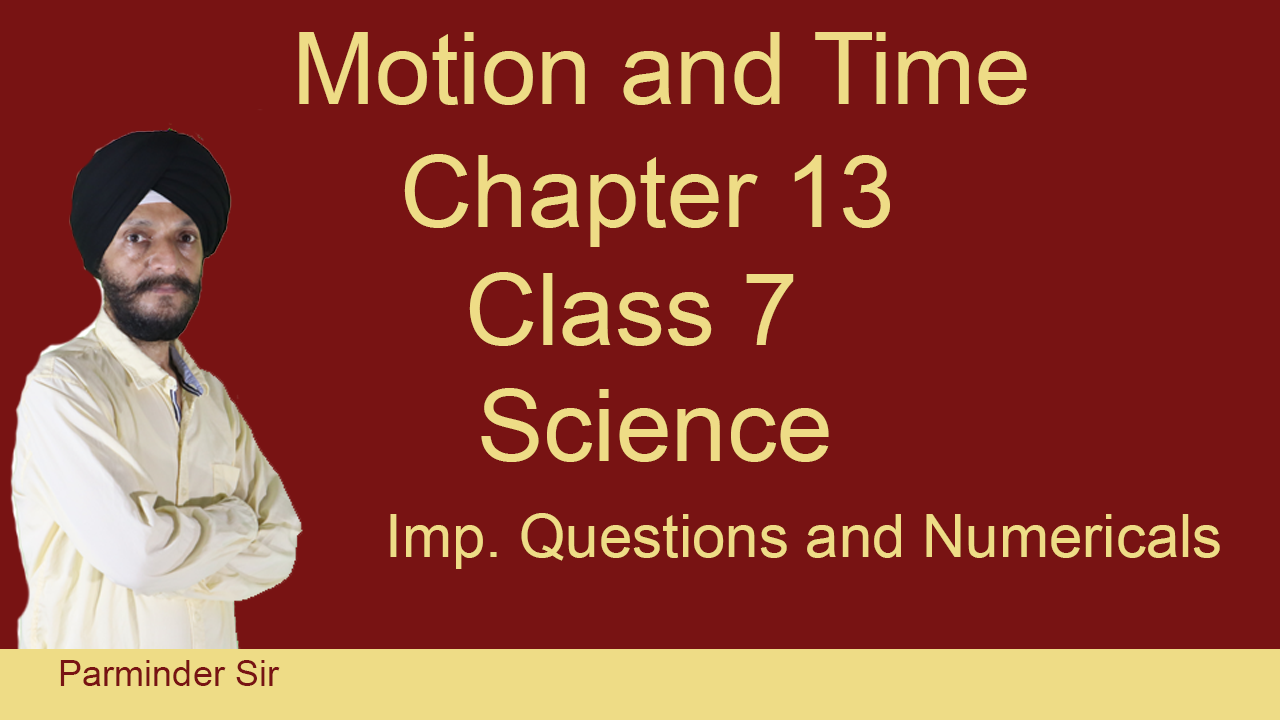### Motion and Time Chapter 13 Class 7 SciencePrabh Kirpa Classes

# Motion and Time Chapter 13 Class 7 Science

Motion and Time Chapter 13 Class 7 ScienceUpdated on Sep, 2023Language - EnglishPrabh Kirpa ClassesTeaching & Academics,7th Class,PhysicsTraining 5 or more people ?

## Course Description

Q1. In a school athletic meet, a boy ran at a speed of 2m/s and stood first in a 200m race. How much distance is covered by him per second?

Q2. Rahul and Rijul are going on the excursion. They both have to go to the same place and cover a certain distance but in different cars. If Rahul covers a certain distance in one hour and Rijul covers the same distance in two hours. Who travels with a higher speed?

Q.3. A simple pendulum takes 32s to complete 20 oscillations. What is the time period of the pendulum?

Q. 4. The distance between the two stations is 240 km. A train takes 4 hours to cover this distance. Calculate the speed of the train.

Q. 5 The basic unit of speed is:

(i) km/min (ii) m/min

(iii) km/h (iv) m/s

Q6. Define the following terms:

A) Distance

B) Time:

C) Speed:

D) Uniform Motion:

E) Average Speed

Q. 7. Change 20 m/s to km/h

Q.8. Change 54km/h to m/s

Q.9. An electric train is moving at a speed of 30m/s. How much distance will it travel in the 30s?

Q.10. A bus takes 30 minutes to travel a distance of 25 km. Calculate the speed of the bus in km/h?

Q.11. A train is traveling at a speed of 110km/h. How long will it take to complete a journey of 605  km, without any stoppage in between?

Q.12. The time taken for 20 oscillations is 40 seconds. Calculate the time period of a simple pendulum.

Q.13. Plot the graph from the following data:

What is the type of motion shown in the graph?

### Goals

What will you learn in this course:

• Q1. In a school athletic meet, a boy ran at a speed 2m/s and stood first in 200m race. How much distance is covered by him per second?
• Q.3. A simple pendulum takes 32s to complete 20 oscillations. What is the time period of the pendulum?
• Q. 4. The distance between two stations is 240 km. A train takes 4 hours to cover this distance. Calculate the speed of the train.
• Q. 5 The basic unit of speed is: (i) km/min (ii) m/min (iii) km/h (iv) m/s
• Q6. Define the following terms:- A) Distance B) Time : C) Speed: D) Uniform Motion: E) Average Speed
• Q. 7. Change 20 m/s to km/h
• Q.8. Change 54km/h to m/s
• Q.9. An Electric train is moving with a speed of 30m/s. How much distance will it travel in 30s?
• Q.10. A bus takes 30 minutes to travel a distance of 25 km. Calculate the speed of bus in km/h?
• Q.11. A train is travelling at a speed of 110km/h. How long will it take to complete a journey of 605 km, without any stoppage in between?
• Q.12. The time taken for 20 oscillations is 40seconds. Calculate the time period of a simple pendulum.
• Q.13. Plot the graph from the following data: Also answer the following question:- What is the type of motion shown in the graph?## Curriculum

Check out the detailed breakdown of what’s inside the course

Motion and Time Chapter 13 Class 7 Science
5 Lectures
•Introduction 04:21 04:21
•Imp. Questions 1- 5 08:46 08:46
•Imp. Questions 6 - 7 08:42 08:42
•Imp. Questions 8 - 12 06:53 06:53
•Imp. Questions 13 - 18 09:09 09:09

## Instructor DetailsPrabh Kirpa Classes

This channel is purely education based. Our aim is to ensure that the students are able to understand each and every question in an easy way.  We take pride in providing quality educational services to the students of Class VII to Class XI  Science NCERT, English, Computer Solutions, Mathematics & Business Studies.  Our goal is to help the leaders of tomorrow to learn to succeed today.

## Course Certificate

User your certification to make a career change or to advance in your current career. Salaries are among the highest in the world.## Feedbacks

A

Amit Singh Chauhan

Excellent